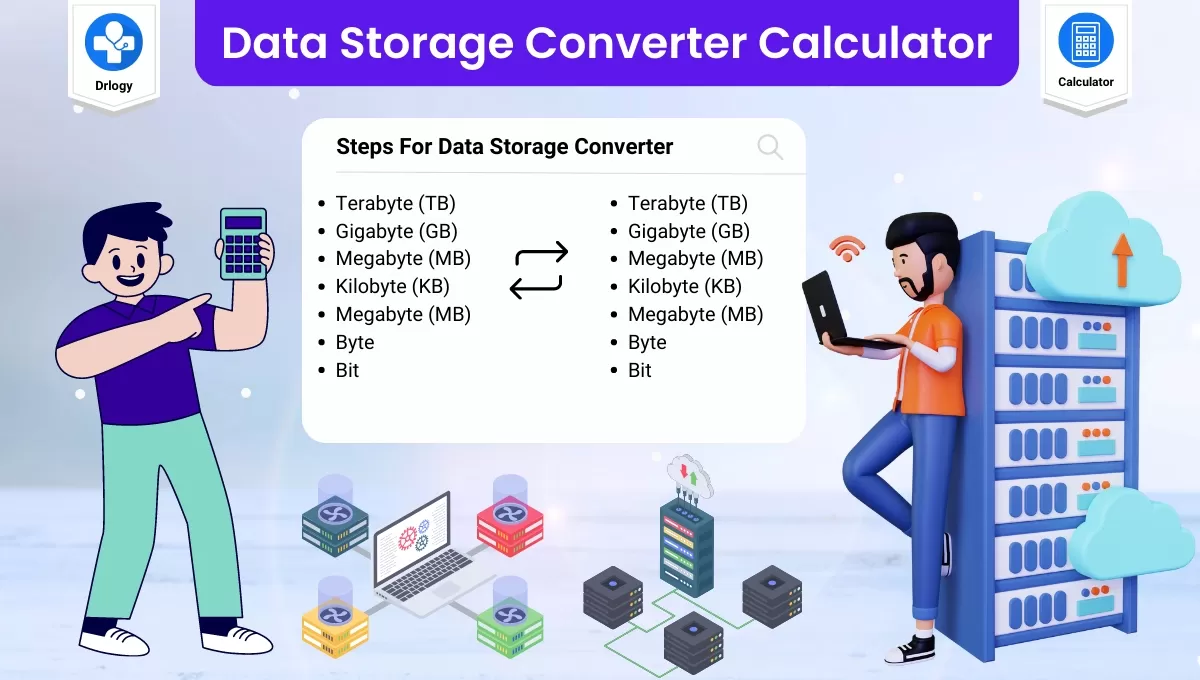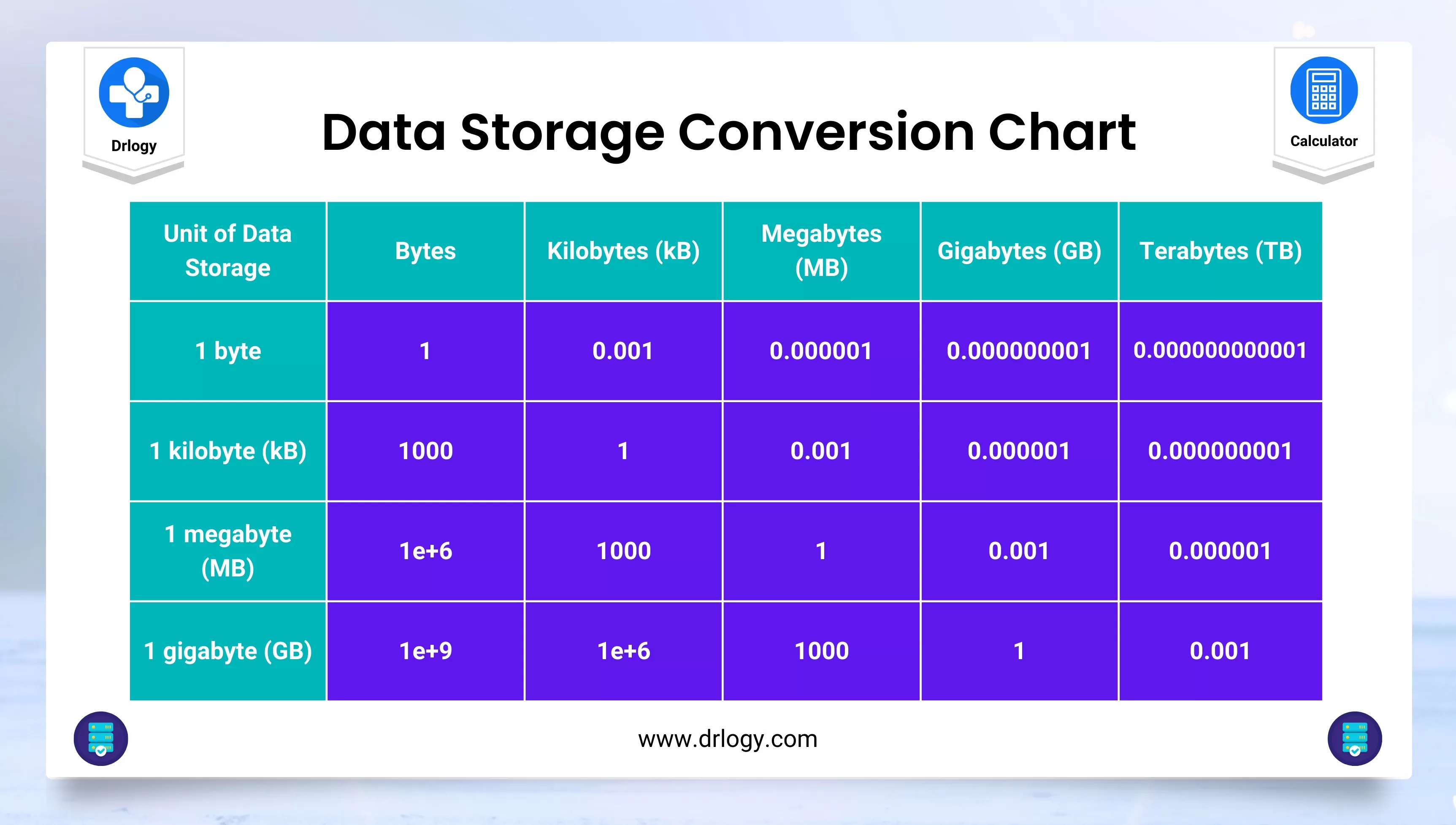• Health
• Pet
• Unit Conversion
• Medical# Data Storage Converter - Data Units Conversion

## Data Storage Converter Calculator

Result : =
keyboard_arrow_down
=
keyboard_arrow_down

## Data Storage Converter Calculator

• A data storage converter calculator is used to convert data from one storage format to another like bite, bytes, kilobyte, megabyte, gigabyte, terabyte, petabyte, exabyte, and more.
• The primary purpose of data storage conversion is to ensure compatibility between different storage systems, devices, or applications.

## Steps For Data Storage Converter Calculator

Here are the general steps for using a data storage converter calculator:

1. Determine the input and output formats: Decide on the format of the data you want to convert and the format you want it to be converted into. For example, you might want to convert binary data into hexadecimal format.
2. Select a data storage converter tool: Choose a data storage converter calculator that supports the input and output formats you need.
3. Input the data: Enter the data you want to convert into the calculator. This can be done by typing the data directly into the input field or uploading a file containing the data.
4. Choose the conversion method: Select the conversion method you want to use. Some calculators offer multiple conversion methods, while others only support one.
5. Verify the output: Check the output data to ensure that it has been converted correctly. If the output is incorrect, check the input data and conversion method to see if there are any errors.
6. Save or export the output: Once you are satisfied with the output data, you can save it to your device or export it to another application or system.

It is important to note that specific data storage converters may have slightly different steps, so it is recommended to refer to the instructions provided by the calculator or tool you are using.

## Data Storage Converter Units Table

Here is a table that shows the most commonly used data storage units and their equivalent values:

Unit Abbreviation Value in Bytes
Bit b 1/8 byte
Byte B 1 byte
Kilobyte KB 1,024 bytes
Megabyte MB 1,048,576 bytes (1024 KB)
Gigabyte GB 1,073,741,824 bytes (1024 MB)
Terabyte TB 1,099,511,627,776 bytes (1024 GB)
Petabyte PB 1,125,899,906,842,624 bytes (1024 TB)
Exabyte EB 1,152,921,504,606,846,976 bytes (1024 PB)
Zettabyte ZB 1,180,591,620,717,411,303,424 bytes (1024 EB)
Yottabyte YB 1,208,925,819,614,629,174,706,176 bytes (1024 ZB)

• It is important to note that some storage manufacturers and software vendors may use different definitions of these units.
• For example, some may use 1,000 bytes as the definition of a kilobyte instead of 1,024 bytes.
• Therefore, it is important to check the specific definitions being used to ensure accurate data storage conversion.## Data Storage Conversion Formula

There are conversion formulas that can be used to convert between data storage units. Here are some of the commonly used formulas:

### Bits To Bytes

To convert from bits to bytes, divide the number of bits by 8:

Bytes = Bits / 8

### Bytes To Kilobytes

To convert from bytes to kilobytes, divide the number of bytes by 1024:

Kilobytes = Bytes / 1024

### KiloBytes To Megabytes

• To convert from kilobytes to megabytes, divide the number of kilobytes by 1024:

Megabytes = Kilobytes / 1024

### Megabytes To Gigabytes

• To convert from megabytes to gigabytes, divide the number of megabytes by 1024:

Gigabytes = Megabytes / 1024

### Gigabytes To Terabytes

• To convert from gigabytes to terabytes, divide the number of gigabytes by 1024:

Terabytes = Gigabytes / 1024

### Terabytes To Petabytes

• To convert from terabytes to petabytes, divide the number of terabytes by 1024:

Petabytes = Terabytes / 1024

These conversion formulas can be used to convert between data storage units for a variety of purposes, such as calculating storage capacity and determining data transfer rates.

## Data Storage Converter Units Benefits

There are several benefits to using a data storage converter calculator, including:

1. Ease of use: A data storage converter calculator makes it easy to quickly convert between different data storage units without the need for manual calculations.
2. Accuracy: Using a calculator ensures accurate conversions, reducing the risk of errors that can occur with manual calculations.
3. Efficiency: A data storage converter calculator can save time by quickly converting large amounts of data, allowing users to focus on other tasks.
4. Flexibility: A calculator can convert between different units of measurement, providing users with the flexibility to work with a variety of data storage formats.
5. Convenience: A calculator is readily available on most devices, making it easy to access and use whenever it is needed.

Summary

Overall, a data storage converter calculator is a useful tool for anyone who works with data storage units and needs to convert between them regularly. Check more unit conversion calculators like this to solve your daily problems on Drlogy Calculator to get exact solution.

Reference

• Data conversion By Wikipedia .
• Memory and Storage Converter .
favorite_border 2156 Likes

## Data Storage Converter Calculator FAQ

### How do I convert a KB file to MB?

To convert a file size in kilobytes (KB) to megabytes (MB), you can use the following formula:

MB = KB / 1024

For example, if you have a file that is 2048 KB in size, you can convert it to MB as follows:

MB = 2048 KB / 1024 = 2 MB

Therefore, the file size is 2 MB.

Note that when converting between units of data storage, it is important to use the correct conversion factor. In this case, we divide by 1024 because there are 1024 kilobytes in a megabyte.

### How do you add MB to GB?

To add megabytes (MB) to gigabytes (GB), you can use the following formula:

1 GB = 1024 MB

Therefore, to add MB to GB, you need to divide the total number of megabytes by 1024 to get the equivalent number of gigabytes. Then, you can add the resulting value to the original number of gigabytes.

For example, let's say you have a file that is 1500 MB in size and you want to add it to another file that is 2.5 GB in size.

• First, convert the size of the file in GB to MB:

2.5 GB = 2.5 x 1024 MB = 2560 MB

• Then, add the two file sizes in MB:

1500 MB + 2560 MB = 4060 MB

• Finally, convert the total size back to GB by dividing by 1024:

4060 MB / 1024 = 3.96 GB (rounded to two decimal places)

Therefore, the total file size is 3.96 GB.

### How do I convert data storage?

To convert data storage from one unit to another, you can use the appropriate conversion factor. The conversion factor depends on the units you are converting between. Here are the steps to convert data storage:

1. Determine the size of the data storage you want to convert.

2. Determine the conversion factor between the units you are converting. For example, to convert from kilobytes (KB) to megabytes (MB), the conversion factor is 1 MB = 1024 KB.

3. Multiply the size of the data storage by the conversion factor to get the equivalent value in the desired unit. For example, to convert 500 KB to MB, you would multiply 500 by 1/1024 (since 1 MB = 1024 KB), which equals approximately 0.488 MB.

4. Round the result to the desired number of decimal places.

Here is an example conversion from gigabytes (GB) to terabytes (TB):

1. Suppose you have a hard drive with a storage capacity of 4.5 GB.

2. The conversion factor between GB and TB is 1 TB = 1024 GB.

3. To convert 4.5 GB to TB, divide by 1024:

4.5 GB / 1024 = 0.00439453 TB

1. Round the result to the desired number of decimal places, for example, to two decimal places:

0.00439453 TB ≈ 0.00 TB (rounded to two decimal places)

Therefore, the storage capacity of the hard drive is approximately 0.00 TB.

View More Health

## Medical Calculator

View More Medical

View More Pet

## Unit Conversion Calculator

View More Unit Conversion

## Financial Calculator

View More Financial
Drlogy®
The Power To Health